# Aggregate Formulas

In reporting the most common data analysis that we do is to apply aggregate functions over the data (like SUM, AVG, COUNT etc.,) and analyze the same. The important aspect to be taken care in such calculations is to compute the aggregation in the context in which the function is applied to provide accurate results. Aggregate Formulas in the Zoho Advanced Analytics app is meant to address this need.

Aggregate Formulas are formulas that you define in Zoho Advanced Analytics, which uses at least one aggregate function (SUM, AVG..) in the calculation. The output of such calculations is always a numeric value (metric). Aggregate formulas are mainly used in creating powerful reports. These formulas can be used in Charts, Pivot Tables, and Summary View report types in the Zoho Advanced Analytics app.

• The result of an Aggregate Formula is always a numeric value.
• Aggregate Formulas will not be added as another column in the base, it is associated with, like Formula Column. But it will be available for report creation when you create a report over the table (listed as part of the columns list).
• The value of an aggregate formula will be computed for each data point that is displayed in the report in which it is used (i.e., the result is not just a single value). This makes it very powerful.
• Aggregate formula can be constructed in a similar way as that of a Formula Column.

The Zoho Advanced Analytics app offers a range of in-built aggregate functions which can be used as part of the Aggregate Formulas. You can construct the formulas using these in-built aggregate functions combining it with the basic arithmetic operators like +, -, / and *.

## Creating Aggregate Formulas

To create an aggregate formula:

1. Open the table to which you want to add the formula column.
2. Select Add > Aggregate Formula option from the toolbar.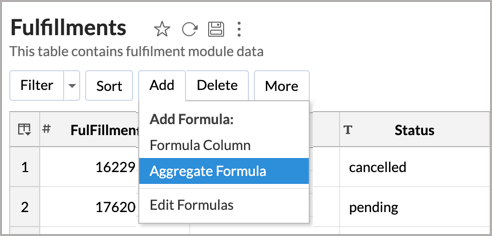Alternatively, you can also right-click on a column in the table and select  Add Formula > Aggregate Formula.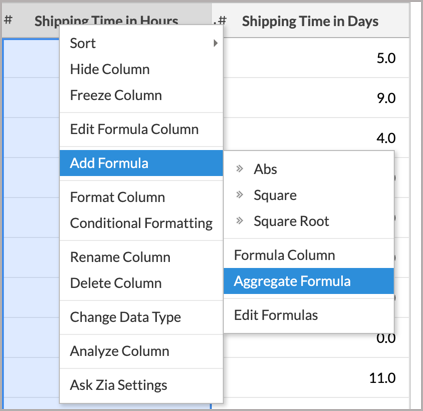3. The Add Aggregate Formula dialog box will open. This dialog box provides easy access to the names of the columns in your table, as well as to all the in-built functions that are available for you to create the formula. A brief description of the function with syntax and an example will be displayed when you hover your mouse over the information icon inline to a function.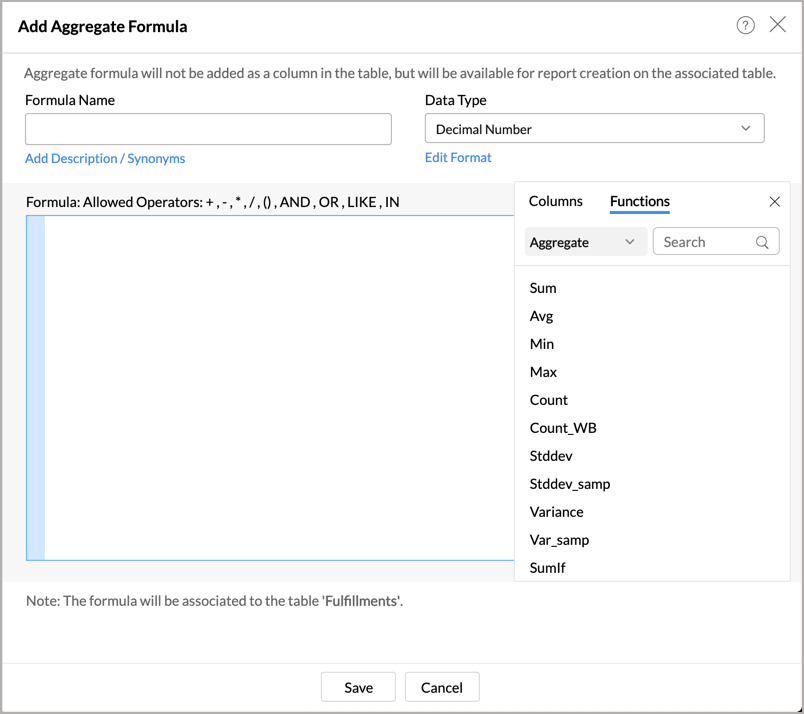4. In the Formula Name text box, type the name that you want to give to the formula.
5. Select the data type of the aggregate result of the formula, using the Data Type drop-down menu. The data type should only be a Numeric value, hence the possible types are Number, Positive Number, Decimal Number, Currency and Percent.
6. In the Formula, text area provide the actual formula. You can select the columns and functions that you want to insert into the formula from the list from the Columns and the Function boxes.

For example, to apply an aggregate formula to calculate average shipping hours the formula could be something like Avg("Fulfillments"."Shipping Time in Hours")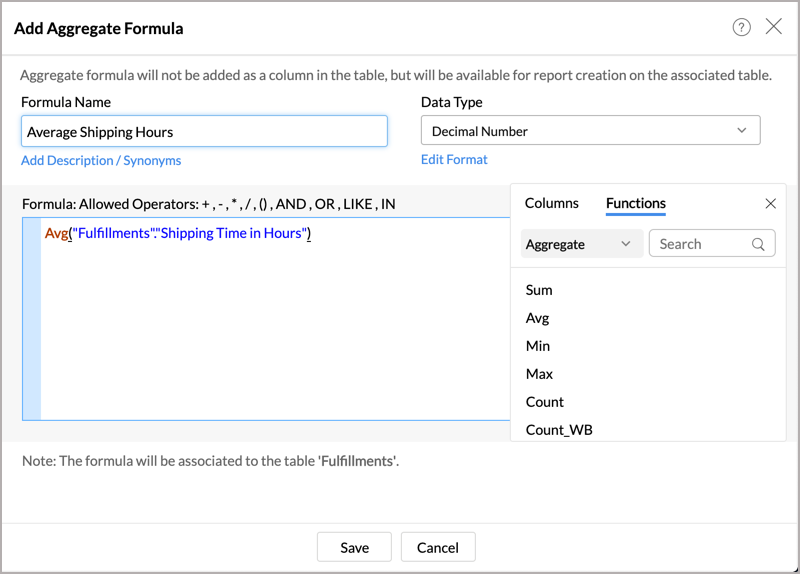7. Click Save.

The result of Aggregate Formulas will not be added as a column in the base data table. It will be listed in the column panel while creating reports. You can access (view) or edit it by clicking Add > Edit Formulas in the toolbar. Read more about viewing & editing aggregate formulas.

### In-built Aggregate Functions

While you create an aggregate formula you can use a wide range of powerful Aggregate functions that are supported by the Zoho Advanced Analytics app. You can use the Formula Column in-built functions, when you create an Aggregate formula, provided it meets the required conditions for an Aggregate formula.

### Creating Aggregate Formula over existing Aggregate Formulas

Zoho Advanced Analytics app enables you to build aggregate formulas using existing aggregate formulas. Reusing existing formulas to create new aggregate formula helps you create powerful formula combinations, as well as eases maintenance of these formula structures in your app.

When you create a new aggregate formula, existing formulas will be listed in the Columns box along with other columns. All the aggregate formulas will be indicated in brown with the symbol. You can insert these formulas into your new formula definition by clicking them, as you do for the other columns in the table. For example, Let's assume that you have already calculated profit percentage and total cost and you want to calculate total profit gained. Then you can use the existing aggregate formulas to calculate total profit gained. The formula could be something like ("Total Cost" * "Profit") / 100.

## Creating Reports using Aggregate Formulas

Aggregate formulas can be used while creating all reports (charts, pivot tables, and summary views) as you would use a column in a table. When you create a new report over the table, in which you have added the aggregate formula, all the formulas will be listed in the Columns list pane on the left, which can be drag & dropped to create the report.

Aggregate formulas can be used in the report as you use any other data columns in the table. The value of the aggregate formula will be computed for each data point that is displayed in the report in which it is used (i.e., the result is not just a single value). In the chart shown in the screenshot below, the average shipping hour aggregate formula is plotted against the shipping city to calculate the average shipping hour per city.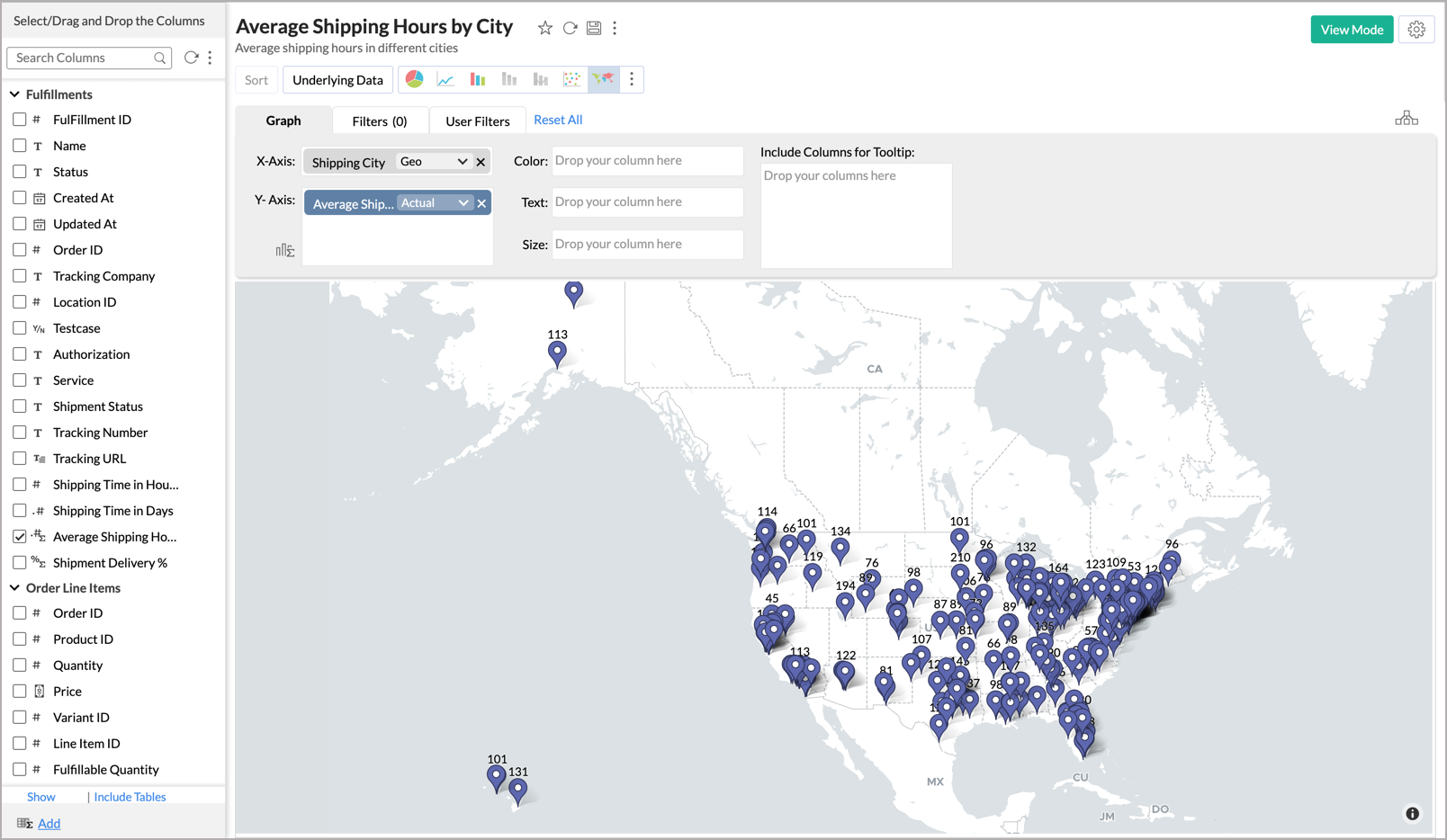When you use an Aggregate formula in a report, you will not be able to apply other functions to the formula (as you do for other columns). The function will always be selected as 'Actual' and cannot be modified.

## View/Edit Formulas

Zoho Advanced Analytics app allows you to view, edit, format, and delete all the formulas defined for a table through the Edit Formula option. You can view & edit both Formula Columns and Aggregate Formulas using this option. To view/edit existing Formula Column or Aggregate Formulas:

1. From the required table, select Add > Edit Formulas option from the toolbar.
2. The Edit Formula dialog box with a list of all the formulas associated with the table will open.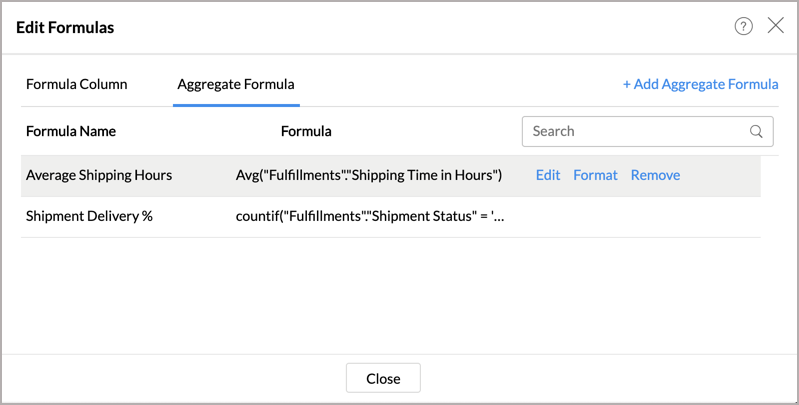3. Navigate to the Aggregate Formula tab.
4. When you mouse over the required formula, you will find the following options. You can modify the existing formula the same way as you would create a new formula.
• Edit Formula: You can edit the constructed formula using the Edit link provided next to the corresponding formula.
• Delete Formula: You can delete an existing Formula any time from the table using the Remove link provided next to the corresponding formula.
• Format Formula: You can change the format of the Formula result, like alignment, decimal places, date format, currency symbols etc., depending on its data type of the formula using the Format link provided. It's similar to how you format a data column.